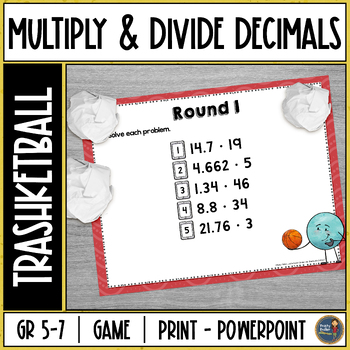# Multiplying and Dividing Decimals Trashketball Math Game5th - 6th
Subjects
Standards
Resource Type
Formats Included
• Zip
Pages
16 (9 color, 7 b/w)

#### Also included in

1. Provide your students with math activities to practice decimals skills. Students will practice skills related to adding decimals, subtracting decimals, multiplying decimals, and dividing decimals. Decimal Operations Bundle includes 12 products. Check out the previews above for each product.Similar R
\$37.00
\$49.50
Save \$12.50
2. Get your students engaged and moving while practicing various math skills. Trashketball is a competitive and motivating math game that involves students solving math problems and shooting baskets at the end of each round.Filetype: Powerpoint✤ The PowerPoint Slides can be uploaded into Google Drive™
\$89.50
\$128.00
Save \$38.50

### Description

Get your students engaged and moving while practicing multiplying decimals and dividing decimals. Multiplying and Dividing Trashketball is a competitive and motivating math game that involves students multiplying and dividing decimals and shooting baskets at the end of each round. There are 4 rounds in this game with 5 questions in each round. This resource includes a PDF & PowerPoint Slides.

✤ The PowerPoint Slides can be uploaded into Google Drive™ and assigned through Google Classroom™. It won't be a game this way but your students will be able to add a text box and answer the questions. ✤

Best of 2020

What's Included:

✢ PDF that includes teacher information, black & white versions (slides, answer keys, rules)

✢ PowerPoint Slides that include rules for students, 4 rounds in color (each on their own slide, NOT EDITABLE), answer keys, and a blank decorative slide to add more rounds if you wish.

Round 1 - multiply decimals and whole numbers

Round 2 - multiply two decimals

Round 3 - divide with a whole number divisor

Round 4 - divide with a decimal divisor

You will need to be able to project the slides for all to see, a large container or trash can, a small ball or bean bag, and some tape to mark off shooting lines.

Similar Resources & Skills:

◈ ◈ ◈ ◈ ◈ ◈ ◈ ◈ ◈ ◈ ◈ ◈ ◈ ◈ ◈ ◈ ◈ ◈ ◈ ◈ ◈ ◈ ◈ ◈ ◈ ◈ ◈ ◈ ◈ ◈ ◈ ◈ ◈ ◈ ◈ ◈ ◈ ◈

➯ Don't forget to leave feedback. You will receive TPT credits that can be used on future purchases!

Total Pages
16 (9 color, 7 b/w)
Included
Teaching Duration
Other
Report this Resource to TpT
Reported resources will be reviewed by our team. Report this resource to let us know if this resource violates TpT’s content guidelines.

### Standards

to see state-specific standards (only available in the US).
Fluently add, subtract, multiply, and divide multi-digit decimals using the standard algorithm for each operation.
Add, subtract, multiply, and divide decimals to hundredths, using concrete models or drawings and strategies based on place value, properties of operations, and/or the relationship between addition and subtraction; relate the strategy to a written method and explain the reasoning used.